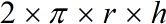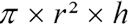Request a Tool

Cylindrical Tank Surface Area Calculator

Cylindrical calculator use to calculate curved surface area and volume of a cylinder.

Area
0
Volume
0

FormulaAreaVolume

• π = a constant value 3.1415
• h = Height of the Cylinder
• r = Radius of the Cylinder

Defination / Uses

A cylinder is a three-dimensional object with two circled ends and a single rectangular sheet connecting them. A curved surface connects the two ends. A cylinder is made up of three parts: two circular ends and a rectangle that is bent around to form the sides.

How to use cylindrical tank surface area calcultor?

Its a single step procedure.

• Add the radius and height values of cylinder in their corresponding fields.

Thats it! You will get your answer in less than a second. Use upper given formulas in case of manual calculation

This type of cylinder is the focus of our surface area of a cylinder calculator. When one of the bases of a cylinder lies directly above the other base, it is said to be right, and when it does not, it is said to be oblique. A cylinder's base can be any plain, closed surface; for example, a circular cylinder has a circular base, whereas a rectangular cylinder has a rectangular base. For quick calculation use our weetools. No sign-up, registration OR captcha is required to use this tool.